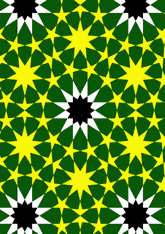data203/P120

## Geometry

• The symmetry group of the tiling is *632 (p6m).
• All the internal angles of the constituent polygons are a multiple of 5°.
• Contains one regular 9-pointed star polygon with vertex angle of 30°.
• Contains one regular 12-pointed star polygon with vertex angle of 60°.
• There are five non-regular reflective tiles (including one kite).
• The tiling satisfies the interlace condition and has no finite interlace and one infinite interlace with straight cross-overs.
• The tiling is edge-to-edge.
• As drawn, contains about 393 polygons.

## References

Publications referenced: